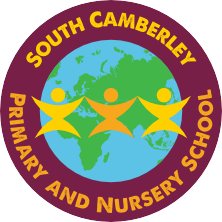# Maths

We teach using the 'Maths No Problem!' scheme in Years 1, 2 and 3 which  is a child centred approach to teaching maths through fun problem solving. There is an emphasis on the use of practical resources to embed concepts before moving on to drawing pictorial representations of their work and finally recording their work. The children follow activities from a task book and then use their own work book to record their learning. The real-life problems encourage higher level thinking and non-routine questions in the work books challenge the children’s understanding.

Topics are revisited at increasingly higher levels as the scheme spirals upwards through the school years.

We aim to introduce Maths No Problem to Year 4, 5 and 6 in September 2020.

The following videos explain some of the fundamental concepts in maths including counting, number bonds, subtraction, mental calculations, multiplication and long division. The final two videos explain how effective the bar model can be in supporting children’s understanding of maths problems at a wide range of levels. The bar model is a way of modelling a maths problem through a picture (or pictorial representation).

Fundmental Idea

If you would like to view this content please

Dr Yeap talks about one of the fundamental ideas in mathematics: that items can only be counted, added, and subtracted if they have the same nouns. He uses a simple example with concrete objects, chocolates and glue sticks to illustrate the point and then shows how it relates to column addition and the addition of fractions.

Number Bonds

If you would like to view this content please

Dr. Yeap explains how young children can use concrete materials and later use pictorial representations as number bonds. Number bonds represent how numbers can be split up into their component parts. Children can explore number bonds using a variety of concrete materials, such as counters with containers and ten frames or with symbols.

Subtraction

If you would like to view this content please

Dr. Yeap explains how standard column subtraction can be taught meaningfully by using children's knowledge of number bonds. Once children can explain how numbers can be split into their component parts, they can adapt their understanding to the conventional column subtraction method.

Mental Calculations

If you would like to view this content please

Dr. Yeap discusses how children can develop an ability to calculate the four operations (addition, subtraction, multiplication and division) in their heads without the use of paper and pencil or calculators.

Multiplication

If you would like to view this content please

Dr. Yeap discusses how children can learn their times tables meaningfully by using visualisation and other strategies.

Mental Calculations

If you would like to view this content please

Dr. Yeap discusses how children can develop an ability to calculate the four operations (addition, subtraction, multiplication and division) in their heads without the use of paper and pencil or calculators.

Long Division

If you would like to view this content please

Dr Yeap discusses how children can learn to do long division meaningfully by first using concrete apparatus, such as base-10 materials, to perform the operations. They can then explore how this idea is represented in the long division algorithm.

Bar Model 1

If you would like to view this content please

Dr. Yeap discusses how diagrams can be used to represent a situation in a problem: such as rectangles representing (unknown) quantities. This method of visualising problems is known as the bar model.

Bar Model 2